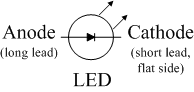EE 212 Lab

Prelab 9: Comparators

1. Recall the operating equation for an op-amp is Vout = A(V+ - V-) where Vout is the output voltage, V+ is the voltage at the non-inverting input, and V- is the voltage at the inverting input. What is the rated gain A of the LM741 and LF356 op-amps in V/V?

2. Given the op-amp gain A, what would one expect Vout to be if V+ > V- (taking saturation into account)?

3. What would one expect Vout to be if V+ < V- (taking saturation into account)?

4. A typical LED allows current to flow in only one direction (from anode to cathode - see figure below), has a voltage drop of around 2V, and requires approximately 10mA of forward bias current to emit light (less current and it will be dim, more current risks damage). Consider the op-amp circuit of figure 1 in the lab. If the output of the op-amp is saturated near either its positive supply voltage (+14V) or negative supply voltage (-14V), what resistor value should be chosen for Rlim such that the current through the LED is 10mA? Hint: does Rlim = (14V - 2V)/10mA or make sense?

5. When Vin = V+ > V- = Vref, which LED (red or green) would you expect to be on?

6. When Vin = V+ < V-= Vref, which LED (red or green) would you expect to be on?

7. Determine the resistor values needed for part two of the lab based upon the criteria given in the lab write-up.